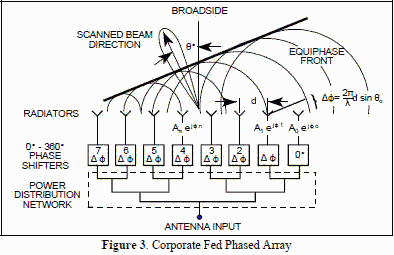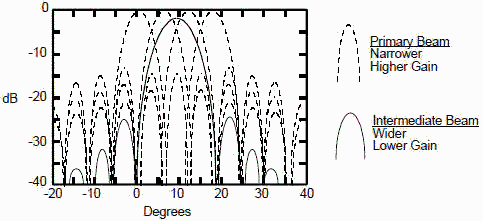# Electronic Warfare and Radar Systems Engineering Handbook- Frequency / Phase Effects of Antennas -

FREQUENCY / PHASE
EFFECTS OF ANTENNAS

The radiation patterns of the antennas presented in the previous section are for antenna geometries most commonly used. The antenna should be viewed as a matching network that takes the power from a transmission line (50 ohm, for example), and matches it to the free space "impedance" of 377 ohms. The most critical parameter is the change of VSWR with frequency. The pattern usually does not vary much from acceptable to the start of unacceptable VSWRs (> 2:1). For a given physical antenna geometric size, the actual radiation pattern varies with frequency.

The antenna pattern depicted in Figure 1 is for the dipole pictured in Section 3-3. The maximum gain is normalized to the outside of the polar plot and the major divisions correspond to 10 dB change. In this example, the dipole length (in wavelengths) is varied, but the same result can be obtained by changing frequency with a fixed dipole length. From the figure, it can be seen that side lobes start to form at 1.258 and the side lobe actually has more gain than the main beam at 1.58. Since the radiation pattern changes with frequency, the gain also changes.Figure 1. Frequency Effects

Figure 2 depicts phase/array effects, which are yet another method for obtaining varied radiation patterns. In the figure, parallel dipoles are viewed from the end. It can be seen that varying the phase of the two transmissions can cause the direction of the radiation pattern to change. This is the concept behind phased array antennas. Instead of having a system mechanically sweeping the direction of the antenna through space, the phase of radiating components is varied electronically, producing a moving pattern with no moving parts. It can also be seen that increasing the number of elements further increases the directivity of the array. In an array, the pattern does vary considerably with frequency due to element spacing (measured in wavelengths) and the frequency sensitivity of the phase shifting networks.Figure 2. Phase / Array Effects

Figure 2 depicts phase/array effects, which are yet another method for obtaining varied radiation patterns. In the figure, parallel dipoles are viewed from the end. It can be seen that varying the phase of the two transmissions can cause the direction of the radiation pattern to change. This is the concept behind phased array antennas. Instead of having a system mechanically sweeping the direction of the antenna through space, the phase of radiating components is varied electronically, producing a moving pattern with no moving parts. It can also be seen that increasing the number of elements further increases the directivity of the array. In an array, the pattern does vary considerably with frequency due to element spacing (measured in wavelengths) and the frequency sensitivity of the phase shifting networks.

Two antennas that warrant special consideration are the phased array and the Rotman bootlace type lens. Both of these antennas find wide application in EW, RADAR, and Communications. The phased array will be described first.

LINEAR PHASED ARRAY

The linear phased array with equal spaced elements is easiest to analyze and forms the basis for most array designs. Figure 3 schematically illustrates a corporate feed linear array with element spacing d.It is the simplest and is still widely used. By controlling the phase and amplitude of excitation to each element, as depicted, we can control the direction and shape of the beam radiated by the array. The phase excitation, ф(n), controls the beam pointing angle, θo, in a phased array. To produce a broadside beam, θo=0, requires phase excitation, ф(n)=0. Other scan angles require an excitation, N(n) = nkd sin(θo), for the nth element where k is the wave number (2π/λ). In this manner a linear phased array can radiate a beam in any scan direction, θo, provided the element pattern has sufficient beamwidth. The amplitude excitation, An, can be used to control beam shape and sidelobe levels. Often the amplitude excitation is tapered in a manner similar to that used for aperture antennas to reduce the sidelobe levels. One of the problems that can arise with a phased array is insufficient bandwidth, since the phase shift usually is not obtained through the introduction of additional path length. However, it should be noted that at broadside the corporate feed does have equal path length and would have good bandwidth for this scan angle.The linear array described above would yield a narrow fan beam with the narrow beamwidth in the plane of the array. To obtain a pencil beam it would be necessary to array several of these line arrays. A problem associated with all electronic scanning is beam distortion with scan angle. Figure 4 illustrates this phenomenon. It results in spread of the beam shape and a consequent reduction in gain known as "scan loss". For an ideal array element, scan loss is equal to the aperture size reduction (projected) in the scan direction which varies as cos θ.

When elements are spaced greater than λ/2 apart, grating lobes are possible when scanning. As the beam is scanned further from broadside, a point is reached at which a second symmetrical main lobe is developed at the negative scan angle from broadside. This condition is not wanted because antenna gain is immediately reduced by 3 dB due to the second lobe. Grating lobes are a significant problem in EW applications because the broad frequency bandwidth requirements mean that at the high end of the frequency band, the elements may be spaced greater than λ/2.

There are many other factors to consider with a phased array such as coning, where the beam curves at large scan angles, and mutual coupling between elements that affect match and excitation. They will not be covered in detail here.

Of interest is the gain of the array which is given by:Where each element is as described in Section 3-4.

Ge(θ) is the element gain which in this case has been taken the same for all elements. Note that if we set A(n)=1, and ф(n)=0, then at broadside where sin(θ) = 0, the gain would be (N Ge). This represents the maximum gain of the array, which typically will not exceed nπ, and is a familiar figure.ROTMAN BOOTLACE LENS

Another method of feeding an array of elements is to use a lens such as the Rotman (rhymes with rotten) Bootlace type shown in Figure 5. The lens consists of a parallel plate region (nowadays microstrip or stripline construction) and cables of specified length connecting the array of elements to the parallel plate region. The geometry of the lens and the cable lengths are designed so that all ray paths traced from a beam port on the right side to its associated wavefront on the left array port side, are equal. This tailoring of the design is accomplished at three focus points (beam ports 1, 4, and 7 in Figure 5). Departure from perfect focus at intermediate beam ports is negligible in most designs.

The Rotman lens provides both true time delay phase shift and amplitude taper in one lens component. The true time delay is one of the distinct advantages of the lens over the phase shifted array since that makes it independent of frequency. To understand how the taper is obtained requires knowledge of the parallel plate region. For a stripline design the unit would consist of a large flat plate-like center conductor sandwiched between two ground planes, and having a shape much like that of the plan view outline shown in Figure 5 with individual tapered launchers (connectors) attached to each beam port and array port. If the antenna is in the receive mode, the energy intercepted on the array port side can be controlled by the angle subtended by the tapered sections of the connector (launcher) much like a larger antenna would intercept a larger portion of energy from free space.

Unlike the phased array with its fine beam steering, the Rotman lens provides only a distinct set of beams. Fine steering is obtained by combining beams either equally or unequally to form intermediate beams. As can be seen in Figure 6, this results in a broader beam with less gain but lower side lobes than the primary beams.

High transmit power can be obtained using a Rotman lens by placing a low power amplifier between each lens output port and its antenna. In this case a separate Rotman lens would have to be used for receiving.Figure 6. Primary and Intermediate Beam Formation in Lens ArraysAbout RF CafeCopyright: 1996 - 2024Webmaster:    Kirt Blattenberger,    BSEE - KB3UON RF Cafe began life in 1996 as "RF Tools" in an AOL screen name web space totaling 2 MB. Its primary purpose was to provide me with ready access to commonly needed formulas and reference material while performing my work as an RF system and circuit design engineer. The Internet was still largely an unknown entity at the time and not much was available in the form of WYSIWYG ... All trademarks, copyrights, patents, and other rights of ownership to images and text used on the RF Cafe website are hereby acknowledged. My Hobby Website:  AirplanesAndRockets.com